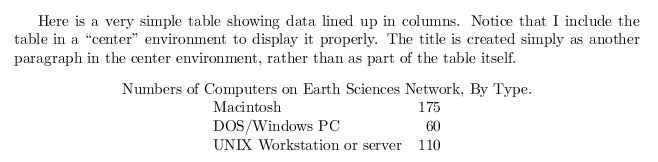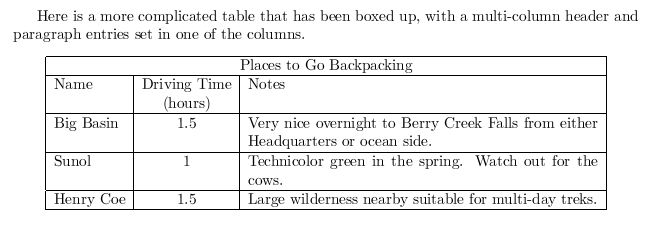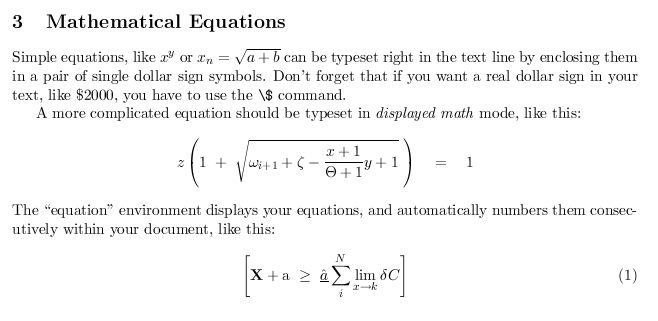## Monday, September 17, 2012

### LaTex Examples

1> Here is a very simple table showing data lined up in columns.
Notice that I include the table in a center'' environment to display
it properly.
The title is created simply as another paragraph in the center environment,
rather than as part of the table itself.
\begin{center}
Numbers of Computers on Earth Sciences Network, By Type.

\begin{tabular}{lr}
Macintosh&175\\
DOS/Windows PC&60\\
Unix Workstation or server&110\\
\end{tabular}
\end{center}2> Here is a more complicated table that has been boxed up, with a multi-column
header and paragraph entries set in one of the columns.
\begin{center}
\begin{tabular}{|l|c|p{3.5in}|}
\hline
\multicolumn{3}{|c|}{Places to Go Backpacking}\\ \hline
Name&Driving Time&Notes\\
&(hours)&\\ \hline
Big Basin&1.5&Very nice overnight to Berry Creek Falls from
either Headquarters or ocean side.\\ \hline
Sunol&1&Technicolor green in the spring. Watch out for the cows.\\ \hline
Henry Coe&1.5&Large wilderness nearby suitable for multi-day treks.\\ \hline
\end{tabular}
\end{center}3> \section {Mathematical Equations}
Simple equations, like $x^y$ or $x_n = \sqrt{a + b}$ can be typeset right
in the text line by enclosing them in a pair of single dollar sign symbols.
Don't forget that if you want a real dollar sign in your text, like \$2000, you have to use the \verb+\$+ command.

A more complicated equation should be typeset in {\em displayed math\/} mode,
like this:
$z \left( 1 \ +\ \sqrt{\omega_{i+1} + \zeta -\frac{x+1}{\Theta +1} y + 1} \ \right) \ \ \ =\ \ \ 1$
The equation'' environment displays your equations, and automatically
numbers them consecutively within your document, like this:
\begin{equation}
\left[
{\bf X} + {\rm a} \ \geq\
\underline{\hat a} \sum_i^N \lim_{x \rightarrow k} \delta C
\right]
\end{equation}

\end{document}